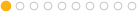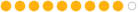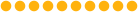### hou.hmath.noise1d returns 0.5

4511   5   1Member23 posts
Joined: July 2008
Offline
I'm trying to use the python noise function.

hou.hmath.noise1d(hou.Vector3(1.0,1.0,1.0))

but it returns 0.5 no matter the input. Am I doing something wrong?

ThanksMember678 posts
Joined: July 2005
Offline
Reason for this is the same as for rand() Houdini function. It generate random number but from the same value it always gives you the same output. Change one value of your vector and you will get different output. It's not full random function.Member23 posts
Joined: July 2008
Offline
Hi,

I know how a noise-function works. The whole idea is to get the same “random” number every time at a certain position.

The problem is

hou.hmath.noise1d(hou.Vector3(1.0,1.0,5.0))
hou.hmath.noise1d(hou.Vector3(2.0,1.0,2.0))
hou.hmath.noise1d(hou.Vector3(4.0,1.0,1.0))

all return 0.5

is this function not implemented yet?Member1390 posts
Joined: July 2005
Offline
burnie
Hi,

I know how a noise-function works. The whole idea is to get the same “random” number every time at a certain position.

The problem is

hou.hmath.noise1d(hou.Vector3(1.0,1.0,5.0))
hou.hmath.noise1d(hou.Vector3(2.0,1.0,2.0))
hou.hmath.noise1d(hou.Vector3(4.0,1.0,1.0))

all return 0.5

is this function not implemented yet?

Try hou.hmath.noise1d(hou.Vector3(1.01,1.01,5.01))

There must be reason for that, noise function in hscript behaves the same way. Don't ask me whyskk.Staff799 posts
Joined: July 2006
Offline
SYmek
There must be reason for that, noise function in hscript behaves the same way. Don't ask me whyThe results of “exhelp noise” do a pretty good job of explaining Perlin noise:

The noise is calculated on a unit-spaced lattice and then internal
points are interpolated from lattice points. This means the noise
will be independent between points one unit distance apart. You can
scale the inputs to achieve different frequencies.

Basically this means that the noise will always be zero at integer values and smoothly varying in-between. Noise1d is just shifted so that 0.5 is the median value.

Here is a CHOPs graph of 1D noise vs time. It always crosses 0.5 at integer time values:

Attachments:
noise_graph.png (38.2 KB)Member23 posts
Joined: July 2008
Offline
Off course! Period of one… now I feel like an idiotThanks for pointing that out. And also, thanks for the quick response!